# [织梦教程] dedecms织梦内容详细页{dede:pagebreak/}分页标签

• TA的每日心情擦汗
2 小时前
• 签到天数: 1370 天

[LV.10]论坛老兵

7195主题 5万积分

管理员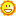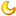魔扣币
217868
贡献
24146
威望
18398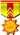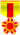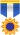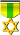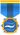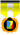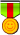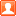发表于 3 天前 | 显示全部楼层 |阅读模式
魔扣币兑换比例：【 50以下 ： ￥1 = 10 魔扣币 】丨【 50 - 100 ：￥1 = 20 魔扣币】丨【 100以上：￥1 = 30 魔扣币 】

### 本帖子中包含更多资源

x
今天在给一个织梦CMS客户定制源码时遇到一个关于内容详细页 和图片集织梦CMS分页过多 导致布局不美观的问题！

经过一翻修改 终于搞定！

让织梦CMS内容和图集分页和栏目页分页一样展示：

具体修改办法如下

首先找到：include/arc.archives.class.php 并且打开。

第一步查找以下代码

function GetPagebreakDM(\$totalPage,\$nowPage,\$aid)
然后从这句代码往下查看 找到  for(\$i=1;\$i<=\$totalPage;\$i++) 替换为以下代码 \$total_list=10; if(\$nowPage >= \$total_list) { \$i = \$nowPage-4; \$total_list = \$nowPage+4; if(\$total_list >= \$totalPage) \$total_list = \$totalPage; }else{ \$i=1; if(\$total_list >= \$totalPage) \$total_list = \$totalPage; } for(\$i;\$i<=\$total_list;\$i++)
第二步查找以下代码

function GetPagebreak(\$totalPage,\$nowPage,\$aid)
和第一步相同 从这句代码往下查看 找到 for(\$i=1;\$i<=\$totalPage;\$i++) 替换为以下代码 \$total_list=10; if(\$nowPage >= \$total_list) { \$i = \$nowPage-4; \$total_list = \$nowPage+4; if(\$total_list >= \$totalPage) \$total_list = \$totalPage; }else{ \$i=1; if(\$total_list >= \$totalPage) \$total_list = \$totalPage; } for(\$i;\$i<=\$total_list;\$i++)
到此 已经全部修改完成  以下是对此修改的一些详解

首先大家会有些疑惑 第一步和第二步好像没什么区别  其实是小有区别的  第一步是修改动态页输出方式  第二步是修改静态页输出方式 所以 大家必须 按照以上两部做出修改

再看看这个 \$total_list=10; 代码，意思就是以10页为一展示 当分页超过10页时 显示效果就是： 上一页 1 2 3 4 5 6 7 8 9 10 下一页

会员购买：>> 点击购买 << | 魔扣币购买：>> 点击购买 <<
承接业务：服务器代维丨网站托管丨SEO
联系客服：微信：morko-net | QQ：1367681973

该用户从未签到

3主题 367积分

中级魔扣魔扣币
182
贡献
182
威望
0发表于 前天 11:16 来自手机 | 显示全部楼层
 生是魔扣的人，死是魔扣的鬼，我愿为魔扣源码论坛添砖加瓦~
回复 支持 反对
 本版积分规则 回帖后跳转到最后一页
•